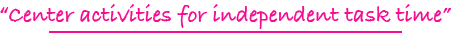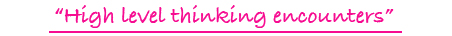•••5 - 8

### ASSESSING MATH UNDERSTANDING AND CONCEPTS

performance, application, and mathematical abstraction tasks for 30 concepts such as factors, multiples, graphs, square numbers, area, perimeter; ideal for assessment

\$15.00

3 - 6

### PROVE IT!: THE "DOING" OF MATHEMATICAL CONCEPTS

50 performance tasks for elementary mathematical concepts such as the commutative property in addition, the understanding of an equation as a balance, and equivalent multiplication expressions; ideal for assessment

\$15.00